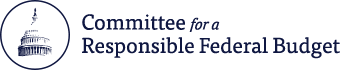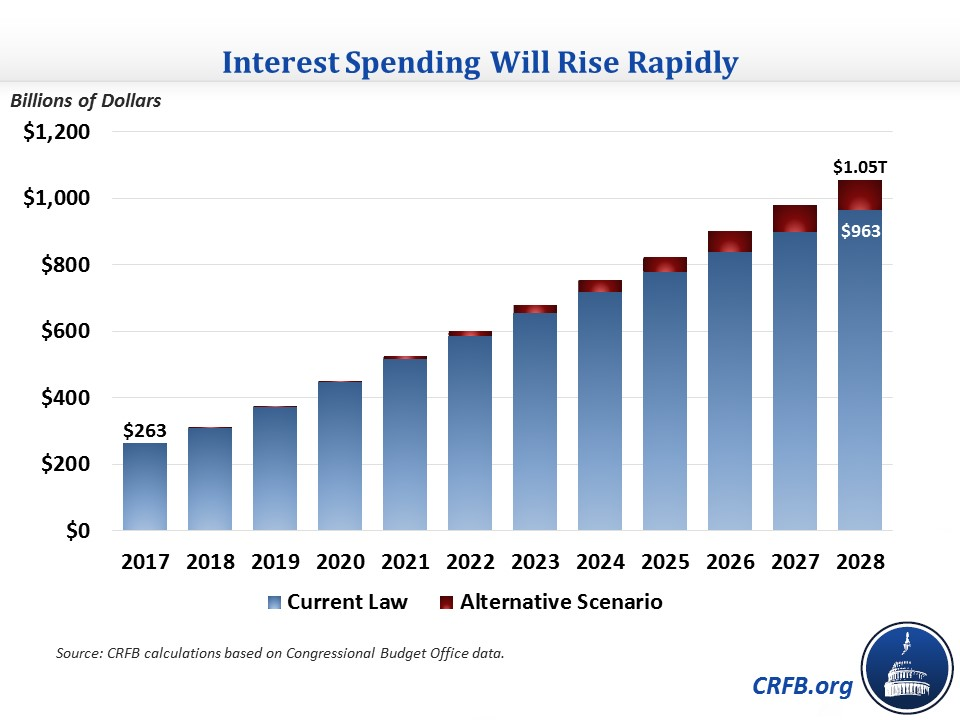# Rising Rates Could Further Balloon Interest Spending

Mar 21, 2018 | Budgets & Projections

Note (5/15/2018): This blog was published before CBO's April 2018 baseline, which provides official estimates of the amount of interest that will be spent over the next ten years. For a discussion of the updated estimates, read here.

The Federal Open Market Committee just announced its decision to further raise the federal funds rate by 0.25 percentage points to 1.5-1.75 percent. As interest rates rise, whether due to Federal Reserve action or other changes in the economy, they will have a significant effect on the budget.

We recently estimated that under current projections, rising debt and interest rates will lead the federal government to spend \$6.8 trillion on interest costs over the next decade. If interest rates end up just 1 percentage point higher than projected, interest costs would increase by a further \$2 trillion. If interest rates return to their pre-recession levels, costs could rise by \$3.4 trillion. These added costs would increase debt to 107 or 112 percent of GDP under current law in 2028.

Projected Interest Rates and Costs

As recently as 2016, the three-month Treasury rate stood at a low of 0.2 percent and the ten-year Treasury rate was at a low of 1.4 percent (and an annual average of 1.8 percent). Already, those rates have increased to 1.7 and 2.9 percent, respectively, and nearly all economists expect them to grow further. Last June, CBO projected the three-month Treasury rate will increase to an ultimate rate of 2.8 percent while the ten-year Treasury rate will increase to 3.7 percent.

These projected interest rates are notably below historic levels. Prior to the recession, between 1990 and 2007, the three-month interest rate averaged 4.2 percent, and the ten-year interest rate averaged 5.8 percent. Most experts believe we will remain below these levels due to slower productivity growth, greater income inequality, higher demand for safe assets, higher foreign capital inflows, and generally observed reductions in global interest rates, but low interest rates are by no means a given.Under CBO's interest rate assumptions, we recently estimated interest costs will quadruple in a decade or so. In a recent analysis, we projected interest will rise from \$263 billion (1.4 percent of GDP) in 2017 to \$965 billion (3.3 percent of GDP) in 2028 under current law and to \$1.05 trillion (3.6 percent of GDP) in 2028 assuming various expiring policies are extended. In either of these scenarios for 2028 interest costs (in percent of GDP) would represent a historic record.The Cost of Higher Interest Rates

While CBO's June 2017 interest rate projections remain plausible, the recent increase in projected federal debt and rise in global growth suggests a possibility that interest rates will be higher. With such a high stock of federal debt, even small increases in interest rates could lead to large increases in interest costs.

Under our baseline projections, we estimate interest costs will total \$6.8 trillion over a decade and \$965 billion in 2028. Based on CBO rules of thumb, we estimate that a just 1 percentage point annual increase in interest rates above CBO's projection would increase interest costs by \$2 trillion (to \$8.8 trillion) over a decade and by \$325 billion (to \$1.3 trillion) in 2028.

If interest rates further increased to their pre-recession (1990-2007) average, interest costs would increase by about \$3.4 trillion (to \$10.1 trillion) over a decade and by \$550 billion (to \$1.5 trillion) in 2028. Under that scenario, interest costs in 2028 would total 5.2 percent of GDP out of a total deficit of 7.6 percent of GDP.Of course, these higher interest payments would mean higher debt. Under current law using CBO's interest projections, we project the debt-to-GDP ratio will rise from 77 percent of GDP today 101 percent of GDP by 2028. If interest rates were one percentage point higher, debt would reach about 107 percent of GDP. If they ultimately reached their pre-recession averages, debt would total 112 percent of GDP. The historical record level of debt held by the public as a percent of GDP for the United States is 106 percent, set just after World War II.Sadly, even these projections may understate the cost of higher interest rates because they assume future policymakers forgo or pay for any extensions of expiring tax and spending legislation. Under our alternative scenario that assumes they do not, debt will rise to 113 percent of GDP and interest payments to \$1.05 trillion (3.6 percent of GDP) by 2028 under CBO's interest rate projections. Very roughly, interest costs could increase by \$4 trillion over ten years, and debt could reach 125-130 percent of GDP in 2028 if interest rates returned to their pre-recession averages on top of our alternative scenario.

Importantly, none of this is to say that higher than expected interest rates are inevitable or even highly likely. But interest rates are at least as likely to be above projections as below, and carrying a lot of debt can leave the federal government more exposed to higher interest rates. Recent legislation has only made that problem worse. Lawmakers need to reverse course to reduce the interest burden and the exposure to eventual higher interest rates.